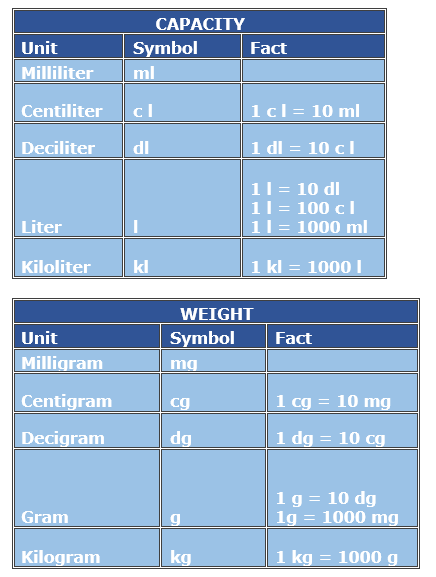# Metric Mass or Capacity Conversion with Whole Number Values

The basic metric units are grams for mass or weight, and liters for volume or capacity. In metric system of units, different units convert into one another rather easily, with one milliliter equaling one cubic centimeter and one gram being the weight of one cubic centimeter of water.

Milligrams and milliliters are small units of weight and capacity respectively.

Pharma drugs and pharma liquids are usually measured in milligrams and milliliters.

Bigger units of weight and capacity are centigrams and centiliters and then grams and liters. 1 gram = 1000 milligrams and 1 liter = 1000 milliliters.

Still bigger units are kilograms and kiloliters.

1 kilogram = 1000 grams; 1 kiloliter = 1000 liters

The tables below help in converting between these various metric units of weight and capacity.Fill in the blank to make the conversion true.

57 kilogram = ____grams

### Solution

Step 1:

1 kg = 1000 gm

Step 2:

57 kg = 57 × 1000 = 57000 gm

So, 57 kg = 57000 gm

Fill in the blank to make the conversion true.

2000 milliliters = ____liters

### Solution

Step 1:

1000 milliliter = 1 liter; 1 ml = 1/1000 l

Step 2:

2000 ml = 2000 × 1/1000 l = 2 liters

So, 2000 ml = 2 liters

Fill in the blank to make the conversion true.

5000 milligram = ____gram

### Solution

Step 1:

1000 mg = 1 g; 1 mg = 1/1000 g

Step 2:

5000 mg = 5000 × 1/1000 = 5 g

So, 5000 mg = 5 g